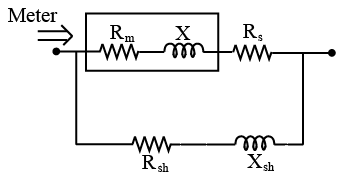Question 183

# An AC meter of resistance $$R_m$$ and resistance $$X_m$$ is connected in series with a resistance $$R_s$$. A shunt of impedance $$(R_{sh} + jX_{sh})$$ is applied in parallel to the existing combination of meter and $$R_s$$. The current division across the two branches will be independent of frequency when• Free SSC Study Material - 18000 Questions
• 230+ SSC previous papers with solutions PDF